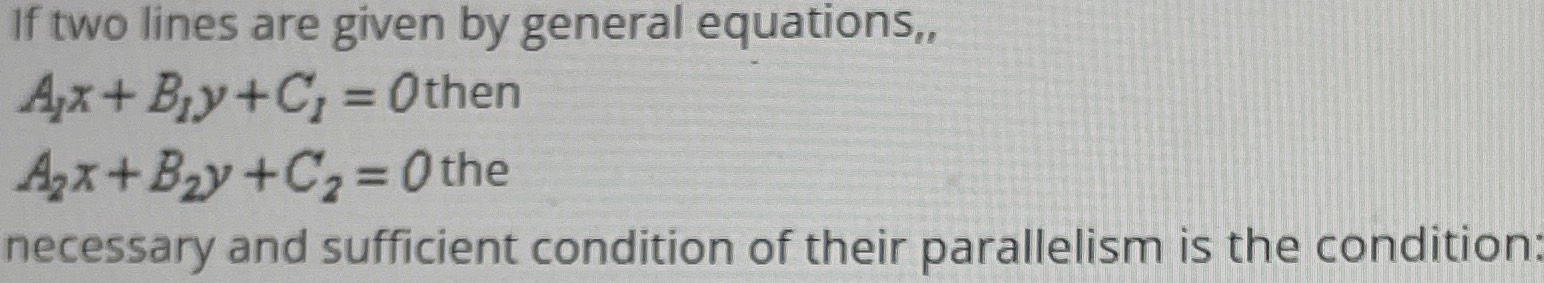### ¿Todavía tienes preguntas de matemáticas?

Pregunte a nuestros tutores expertos
Algebra
PreguntaIf two lines are given by general equations,,

$$A _ { 1 } x + B _ { 1 } y + C _ { 1 } = O$$ then

$$A _ { 2 } x + B _ { 2 } y + C _ { 2 } = O$$ the necessary and sufficient condition of their parallelism is the condition:

$$\frac{A_{1} }{B_{1} } = \frac{A_{2} }{B_{2} }$$(B$$_{1}$$≠0, B$$_{2}$$≠0) or B$$_{1}$$=B$$_{2}$$=≠0